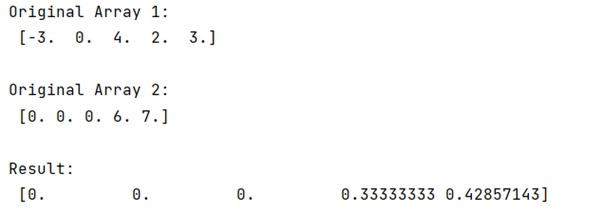# NumPy: How to return 0 with divide by zero?

Learn, how to return 0 with divide by zero?
Submitted by Pranit Sharma, on December 24, 2022

NumPy is an abbreviated form of Numerical Python. It is used for different types of scientific operations in python. Numpy is a vast library in python which is used for almost every kind of scientific or mathematical operation. It is itself an array which is a collection of various methods and functions for processing the arrays.

## Returning 0 with divide by zero

Here, we need to return 0 with divide by zero – that means we need to perform and element-wise division but if a zero is encountered we need the quotient just to be zero.

For this purpose, we could have used a simple for loop over the array but it will result in a loss of optimization. Hence, we need some sort of divide function to return zero upon dividing by zeroes instead of getting errors.

NumPy provides us with a technique of the 'where' clause, we will use numpy.divide() method where we will pass the arrays and also, we will pass an argument called out to define what the result should look like when a zero is encountered.

Let us understand with the help of an example,

## Python code to return 0 with divide by zero

```# Import numpy
import numpy as np

# Creating numpy arrays
arr1 = np.array([-3, 0, 4, 2, 3], dtype=float)
arr2 = np.array([ 0, 0, 0, 6, 7], dtype=float)

# Display original arrays
print("Original Array 1:\n",arr1,"\n")
print("Original Array 2:\n",arr2,"\n")

# Defining an expression
# for division of arrays
res = np.divide(arr1, arr2, out=np.zeros_like(arr1), where=arr2!=0)

# Display result
print("Result:\n",res)
```

Output: